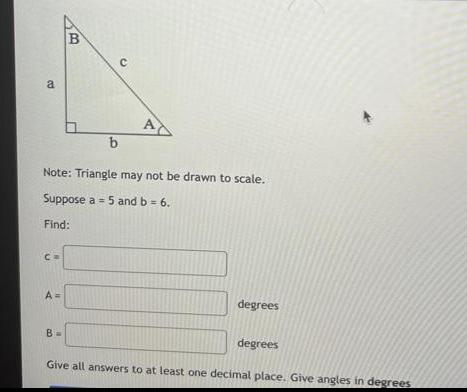Question:

# Note: Triangle may not be drawn to scale. Suppose a = 5 and

Last updated: 7/30/2022Note: Triangle may not be drawn to scale. Suppose a = 5 and b = 6. Find: C A degrees degrees Give all answers to at least one decimal place. Give angles in degrees